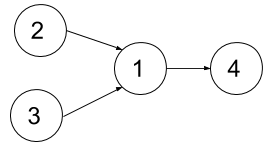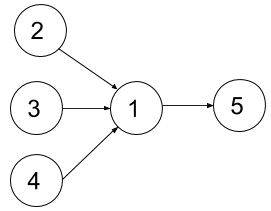1494. Parallel Courses II

Given the integer `n` representing the number of courses at some university labeled from `1` to `n`, and the array `dependencies` where `dependencies[i] = [xi, yi]`  represents a prerequisite relationship, that is, the course `xi` must be taken before the course `yi`.  Also, you are given the integer `k`.

In one semester you can take at most `k` courses as long as you have taken all the prerequisites for the courses you are taking.

Return the minimum number of semesters to take all courses. It is guaranteed that you can take all courses in some way.

Example 1:```Input: n = 4, dependencies = [[2,1],[3,1],[1,4]], k = 2
Output: 3
Explanation: The figure above represents the given graph. In this case we can take courses 2 and 3 in the first semester, then take course 1 in the second semester and finally take course 4 in the third semester.
```

Example 2:```Input: n = 5, dependencies = [[2,1],[3,1],[4,1],[1,5]], k = 2
Output: 4
Explanation: The figure above represents the given graph. In this case one optimal way to take all courses is: take courses 2 and 3 in the first semester and take course 4 in the second semester, then take course 1 in the third semester and finally take course 5 in the fourth semester.
```

Example 3:

```Input: n = 11, dependencies = [], k = 2
Output: 6
```

Constraints:

• `1 <= n <= 15`
• `1 <= k <= n`
• `0 <= dependencies.length <= n * (n-1) / 2`
• `dependencies[i].length == 2`
• `1 <= xi, yi <= n`
• `xi != yi`
• All prerequisite relationships are distinct, that is, `dependencies[i] != dependencies[j]`.
• The given graph is a directed acyclic graph.

1494. Parallel Courses II
``````struct Solution;

struct Solver {
n: usize,
k: usize,
pre: Vec<u32>,
memo: Vec<i32>,
}

impl Solver {
fn new(n: usize, k: usize, pre: Vec<u32>) -> Self {
let mut memo: Vec<i32> = vec![-1; (1 << n) as usize];
memo = 0;
Solver { n, k, pre, memo }
}

fn dfs(&self, k: usize, cur: u32, available: u32, groups: &mut Vec<u32>) {
if k == 0 {
groups.push(cur);
}
if available != 0 && k > 0 {
let bit = 1 << available.trailing_zeros();
self.dfs(k - 1, cur | bit, available & !bit, groups);
self.dfs(k, cur, available & !bit, groups);
}
}

fn dp(&mut self, left: u32) -> i32 {
if self.memo[left as usize] != -1 {
self.memo[left as usize]
} else {
let mut available: u32 = 0;
for i in 0..self.n {
if left & 1 << i != 0 && self.pre[i] & left == 0 {
available |= 1 << i;
}
}
let res = if available.count_ones() <= self.k as u32 {
1 + self.dp(left & !available)
} else {
let mut groups: Vec<u32> = vec![];
self.dfs(self.k, 0, available, &mut groups);
groups
.into_iter()
.map(|g| 1 + self.dp(left & !g))
.min()
.unwrap()
};
self.memo[left as usize] = res;
res
}
}
}

impl Solution {
fn min_number_of_semesters(n: i32, dependencies: Vec<Vec<i32>>, k: i32) -> i32 {
let n = n as usize;
let k = k as usize;
let mut pre = vec![0; n];
for dependency in dependencies {
let x = dependency as usize - 1;
let y = dependency as usize - 1;
pre[y] |= 1 << x;
}
let mut solver = Solver::new(n, k, pre);
solver.dp((1 << n) - 1)
}
}

#[test]
fn test() {
let n = 4;
let dependencies = vec_vec_i32![[2, 1], [3, 1], [1, 4]];
let k = 2;
let res = 3;
assert_eq!(Solution::min_number_of_semesters(n, dependencies, k), res);
let n = 5;
let dependencies = vec_vec_i32![[2, 1], [3, 1], [4, 1], [1, 5]];
let k = 2;
let res = 4;
assert_eq!(Solution::min_number_of_semesters(n, dependencies, k), res);
let n = 11;
let dependencies = vec_vec_i32![];
let k = 2;
let res = 6;
assert_eq!(Solution::min_number_of_semesters(n, dependencies, k), res);
}
``````